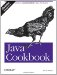# Recipe 5.17 Multiplying Matrices

#### Problem

You need to multiply a pair of two-dimensional arrays, as is common in mathematical and engineering applications.

#### Solution

Use the following code as a model.

#### Discussion

It is straightforward to multiply an array of a numeric type. The code in Example 5-3 implements matrix multiplication.

##### Example 5-3. Matrix.java
`/**   * Multiply two matrices.   * Only defined for int:   * for long, float, and double.   */  public class Matrix {        /* Matrix-multiply two arrays together.       * The arrays MUST be rectangular.       * @author Tom Christiansen & Nathan Torkington, Perl Cookbook version.       */      public static int[][] multiply(int[][] m1, int[][] m2) {          int m1rows = m1.length;          int m1cols = m1.length;          int m2rows = m2.length;          int m2cols = m2.length;          if (m1cols != m2rows)              throw new IllegalArgumentException(         int[][] result = new int[m1rows][m2cols];            // multiply          for (int i=0; i<m1rows; i++)              for (int j=0; j<m2cols; j++)                  for (int k=0; k<m1cols; k++)                  result[i][j] += m1[i][k] * m2[k][j];            return result;      }        public static void mprint(int[][] a) {          int rows = a.length;          int cols = a.length;          System.out.println("array["+rows+"]["+cols+"] = {");          for (int i=0; i<rows; i++) {              System.out.print("{");              for (int j=0; j<cols; j++)                  System.out.print(" " + a[i][j] + ",");              System.out.println("},");          }          System.out.println(":;");      }  }`

Here is a program that uses the Matrix class to multiply two arrays of ints:

`// MatrixUse.java  int x[][] = {      { 3, 2, 3 },      { 5, 9, 8 },  };  int y[][] = {      { 4, 7 },      { 9, 3 },      { 8, 1 },  };  int z[][] = Matrix.multiply(x, y);  Matrix.mprint(x);  Matrix.mprint(y);  Matrix.mprint(z);`

Consult a book on numerical methods for more things to do with matrices; one of our reviewers recommends Numerical Recipes in Fortran by Teukolsky, Flannery, et al., available as a PDF from http://www.library.cornell.edu/nr/bookfpdf.html. Commercial software packages can do some of these calculations for you, including the Visual Numerics libraries, which can be downloaded from http://www.vni.com.Java Cookbook, Second Edition
ISBN: 0596007019
EAN: 2147483647
Year: 2003
Pages: 409
Authors: Ian F Darwin

Similar book on Amazon